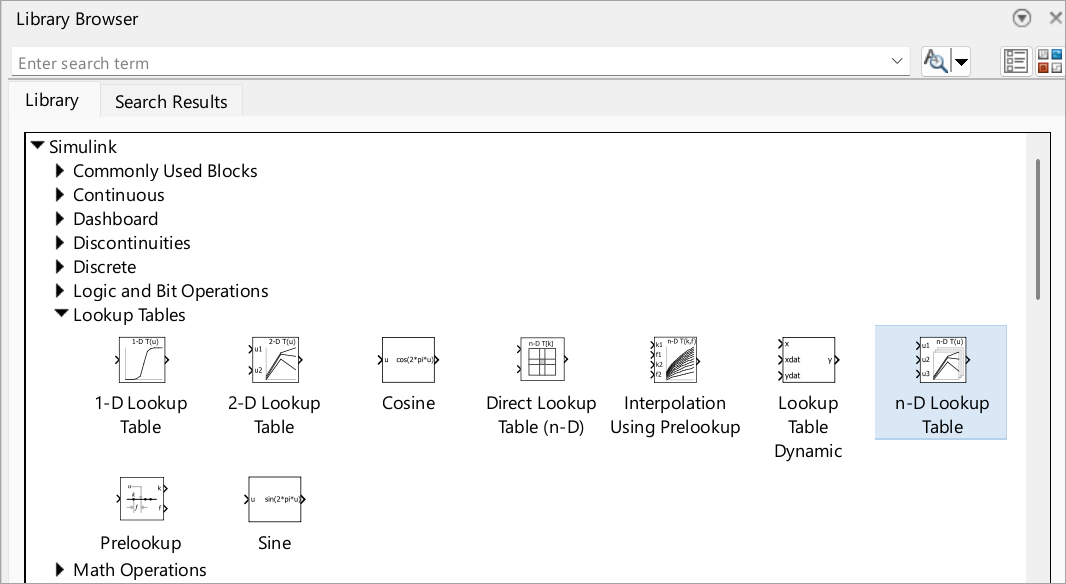Main Content

이 번역 페이지는 최신 내용을 담고 있지 않습니다. 최신 내용을 영문으로 보려면 여기를 클릭하십시오.

# 비선형성

룩업 테이블로 비선형성 모델링, 입력값을 출력값에 매핑하여 수학 함수 근사

비선형성 모델링에 익숙하지 않은 경우 먼저 Lookup Table 블록을 사용해 보십시오. Lookup Table 블록은 데이터 배열을 사용해 입력값을 출력값에 매핑하여 수학 함수를 근사합니다. N개 변수에서 함수를 근사하려면 n-D Lookup Table 블록을 사용하십시오.## 블록

 1-D Lookup Table Approximate one-dimensional function 2-D Lookup Table Approximate two-dimensional function Direct Lookup Table (n-D) Index into n-dimensional table to retrieve element, vector, or 2-D matrix Interpolation Using Prelookup Use precalculated index and fraction values to accelerate approximation of N-dimensional function Lookup Table Dynamic Approximate a one-dimensional function using dynamic table n-D Lookup Table Approximate n-dimensional function Prelookup Compute index and fraction for Interpolation Using Prelookup block Sine, Cosine Implement fixed-point sine or cosine wave using lookup table approach that exploits quarter wave symmetry

## 클래스

 `Simulink.LookupTable` Store and share lookup table and breakpoint data, configure the data for ASAP2 and AUTOSAR code generation `Simulink.Breakpoint` Store and share data for a breakpoint set, configure the data for ASAP2 and AUTOSAR code generation `Simulink.lookuptable.Evenspacing` Configure even spacing set data for lookup table object `Simulink.lookuptable.Table` Configure table data for lookup table object `Simulink.lookuptable.Breakpoint` Configure breakpoint set data for lookup table object `Simulink.lookuptable.StructTypeInfo` Configure settings for structure type that lookup table object uses in the generated code

## 예제 및 방법

Enter Breakpoints and Table Data

How to specify breakpoint data sets and table data for lookup table blocks

Edit Lookup Tables

How to change the elements of lookup tables

Import Lookup Table Data from MATLAB

You can import table and breakpoint data from variables in the MATLAB workspace by referencing them in the Table and Breakpoints tab of the dialog box.

Import Lookup Table Data from Excel

This example shows how to use the MATLAB `xlsread` function in a Simulink model to import data into a lookup table.

Create a Logarithm Lookup Table

Example demonstrating how to work with lookup table blocks

Prelookup and Interpolation Blocks

Provides examples of using Prelookup and Interpolation Using Prelookup blocks together

Update Lookup Table Blocks to New Versions

Process for updating existing models to use current versions of lookup table blocks

Optimize Generated Code for Lookup Table Blocks

Provides tips for optimizing generated code for lookup table blocks

View Simulink.LookupTable Object Data Using the Property Dialog Box Tabular Interface

Work with lookup table objects with `Simulink.LookupTable` property dialog box.

## 개념

About Lookup Table Blocks

Brief overview of lookup table blocks

Anatomy of a Lookup Table

Introduces the components of a lookup table

Lookup Tables Block Library

Describes the various blocks in the Lookup Tables library

Guidelines for Choosing a Lookup Table

How to select the best lookup table for your application

Characteristics of Lookup Table Data

Describes requirements of lookup table data and outlines how to represent discontinuous data

Methods for Approximating Function Values

How to set the method by which lookup table blocks approximate function values

## 지원

#### Model-Based Design for Embedded Control Systems

Download white paper